## 【C语言进阶】动态内存管理

article2023/12/9 3:58:22/1.malloc函数

2.free函数

​3.calloc函数

4.realloc函数

1.对NULL指针的解引用

2.对动态开辟空间的越界访问

3.对非动态开辟内存使用free释放

5.对同一块动态内存多次释放

6.动态开辟内存忘记释放(内存泄漏)

# 一.为什么存在动态内存分配？

``````int val = 20;//在栈空间上开辟4哥字节
int arr = { 0 };//在栈空间上开辟10个字节的连续的空间``````

1.空间开辟大小是固定的
2.数组在申明的时候，必须指定数组的长度，它所需要的内存在编译时分配。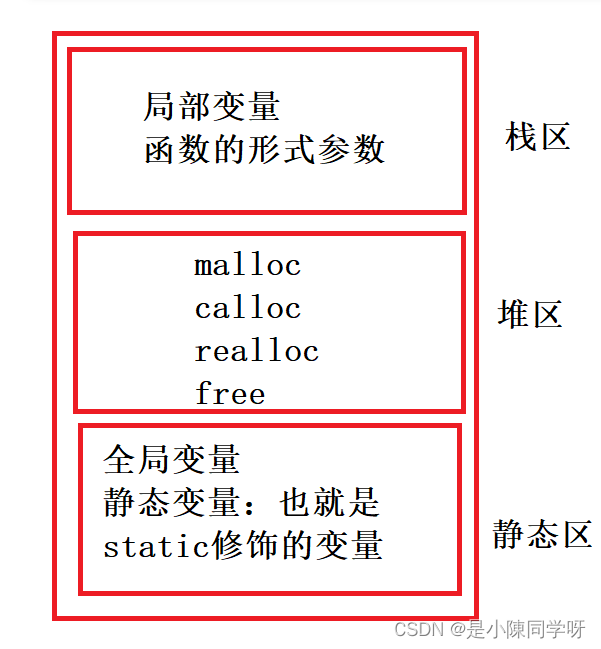# 二.动态内存管理的函数

### 1.malloc函数

C语言提供了一个动态内存开辟的函数：

``void *malloc( size_t size );``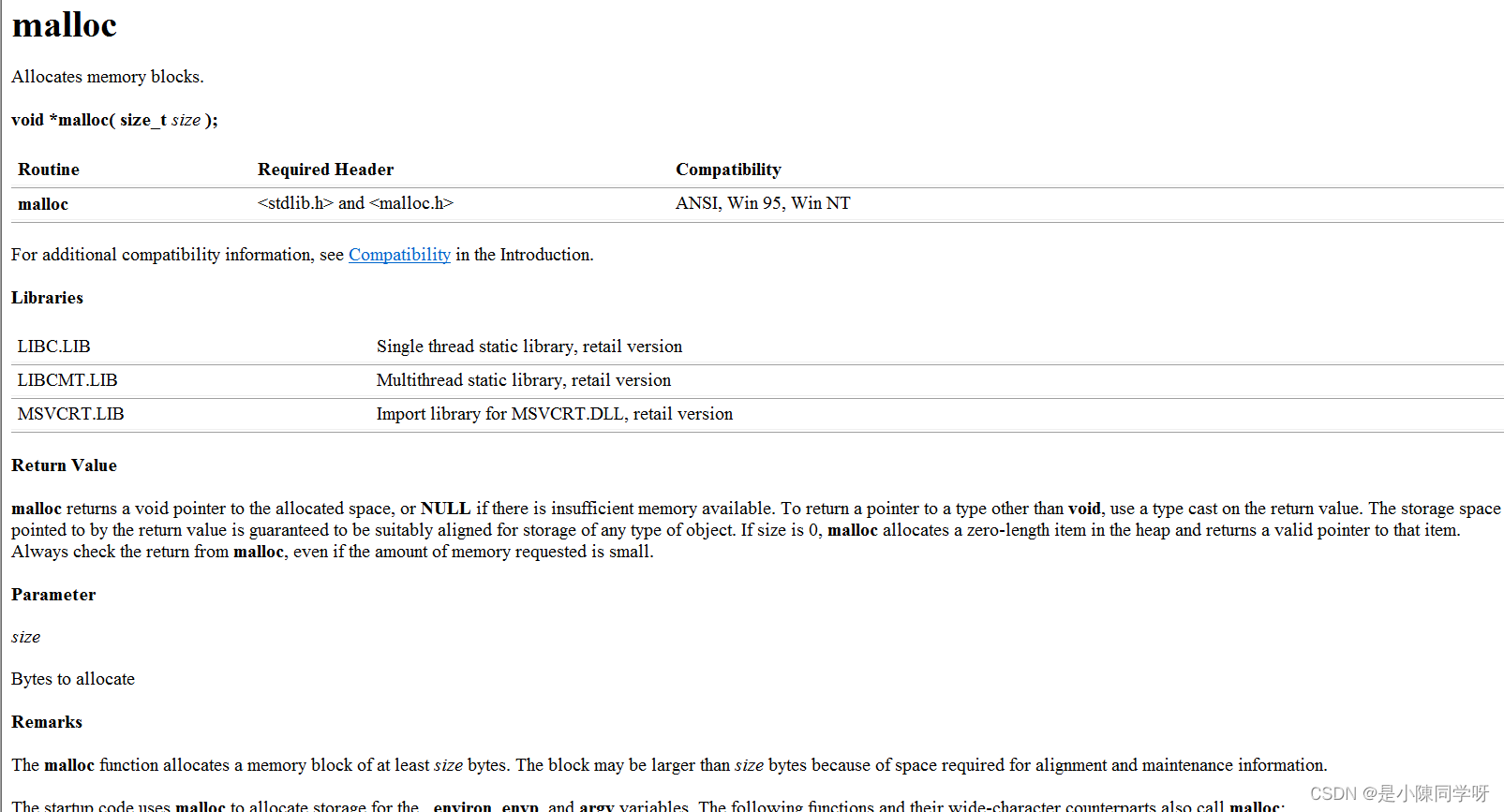这个函数向内存申请一块连续可用的空间，并返回指向这块空间的指针。

●如果开辟成功，则返回一个指向开辟好空间的指针。
●如果开辟失败，则返回一个NULL指针,因此malloc的返回值一定要做检查。
●返回值的类型是void*，所以malloc函数并不知道开辟空间的类型，具体在使用的时候使用者自己来决定。
●如果参数size为0，malloc的行为是标准是未定义的，取决于编译器。

``````#include<stdlib.h>//malloc函数需要的头文件
#include<string.h>//strerror函数需要的头文件
#include<errno.h>//errno需要的头文件
int main()
{
//申请
int* p = (int*)malloc(20);//向内存申请20个字节
if (p == NULL)//这里必须要判断，可能会开辟失败
{
printf("%s\n", strerror(errno));//打印错误信息
return;
}
//释放
free(p);
p = NULL;
return 0;
}``````

``````int main()
{
//申请
int* p = (int*)malloc(200000000000);
if (p == NULL)//这里必须要判断，可能会开辟失败
{
printf("%s\n", strerror(errno));//打印错误信息
return;
}
//释放
free(p);
p = NULL;
return 0;
}``````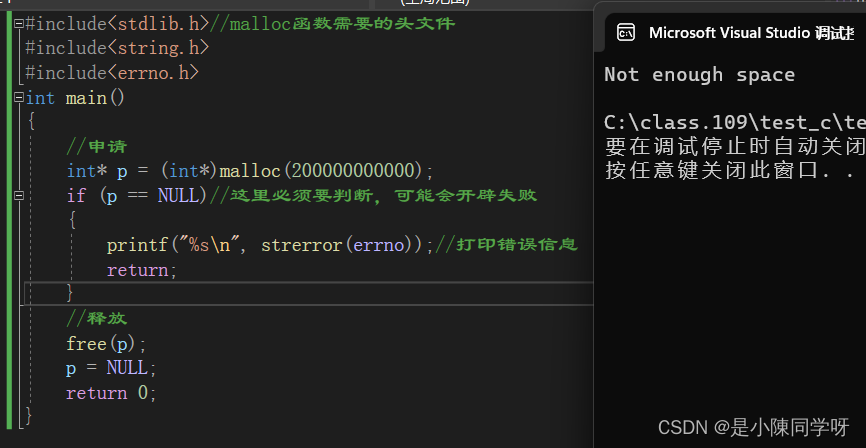### 2.free函数

C语言提供了另外一个函数free, 专门是角来做动态内存的释放和回收的，函数原型如下:

``void free (void* ptr);``

free函数用来释放动态开辟的内存。
●如果参数ptr指向的空间不是动态开辟的，那free函数的行为是未定义的。
●如果参数ptr是NULL指针，则函数什么事都不做。
malloc和free都声明在stdlib.h头文件中。

``````int main()
{

int* p = (int*)malloc(20);
if (p == NULL)
{
printf("%s\n", strerror(errno));
return;
}
int i = 0;
for (i = 0; i < 5; i++)
{
*(p + i) = i + 1;
}
for (i = 0; i < 5; i++)
{
printf("%d ", *(p + i));
}
free(p);//这里必须返回空间给操作系统
p = NULL;//且这里要给开辟的空间赋为NULL
return 0;
}``````

###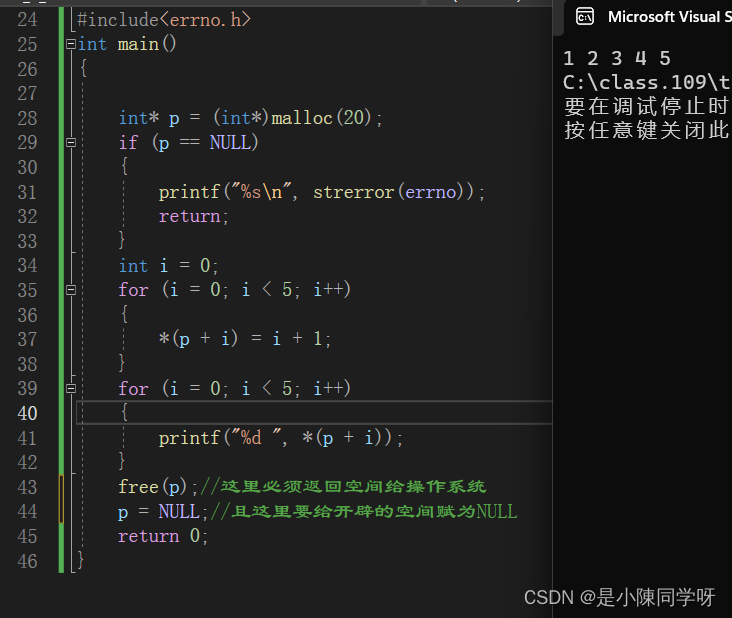3.calloc函数

C语言还提供了一个函数叫calloc，calloc 函数也用来动态内存分配。函数原型如下:

``void* calloc (size_ t num， size_ t size) ;``

●函数的功能是为num个大小为size的元素开辟一块空间， 并且把空间的每个字节初始化为0
●与函数malloc的区别只在于calloc会在返回地址之前把申请的空间的每个字节初始化为全0。

``````int main()
{
int* p = (int*)calloc(10, sizeof(int));//开辟10个大小为int的空间
if (p == NULL)
{
printf("%s\n", strerror(errno));
return;
}
int i = 0;
for (i = 0; i < 10; i++)
{
printf("%d ", *(p + i));
}
free(p);
p = NULL;
return 0;
}``````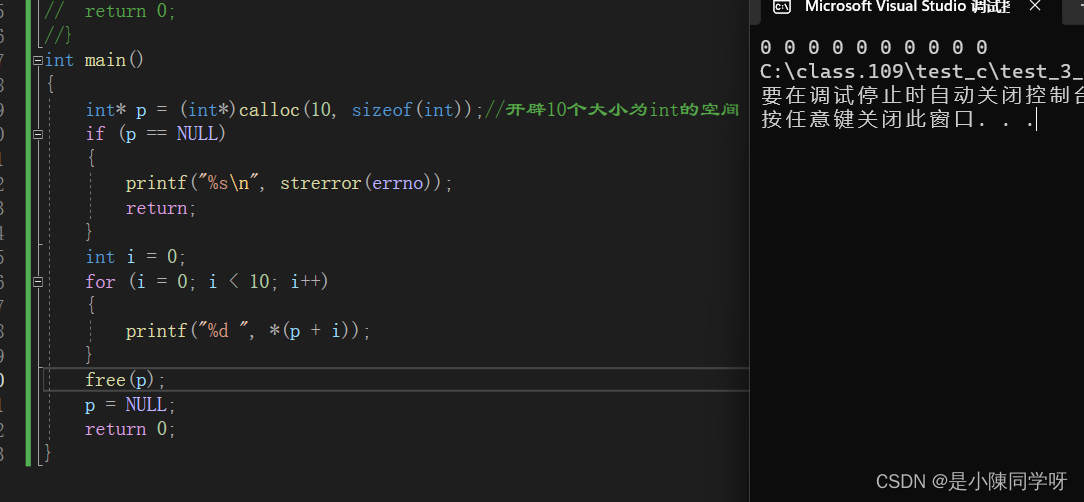这里即使你没有赋值，malloc内部也会自动初始化为0。

calloc和malloc的对比：

1.参数不一样，calloc时两个参数，而malloc是一个参数

2.都是在堆区上面申请内存空间，但是malloc函数不初始化calloc函数会初始化为0

### 4.realloc函数

●realloc函数的出现让动态内存管理更加灵活

``void* realloc (void* ptr, size. _t size);``

●ptr是要调整的内存地址
●size 调整之后新大小.
●返回值为调整之后的内存起始位置。
●这个函数调整原内存空间大小的基础上，还会将原来内存中的数据移动到新的空间。
realloc内部使用规则：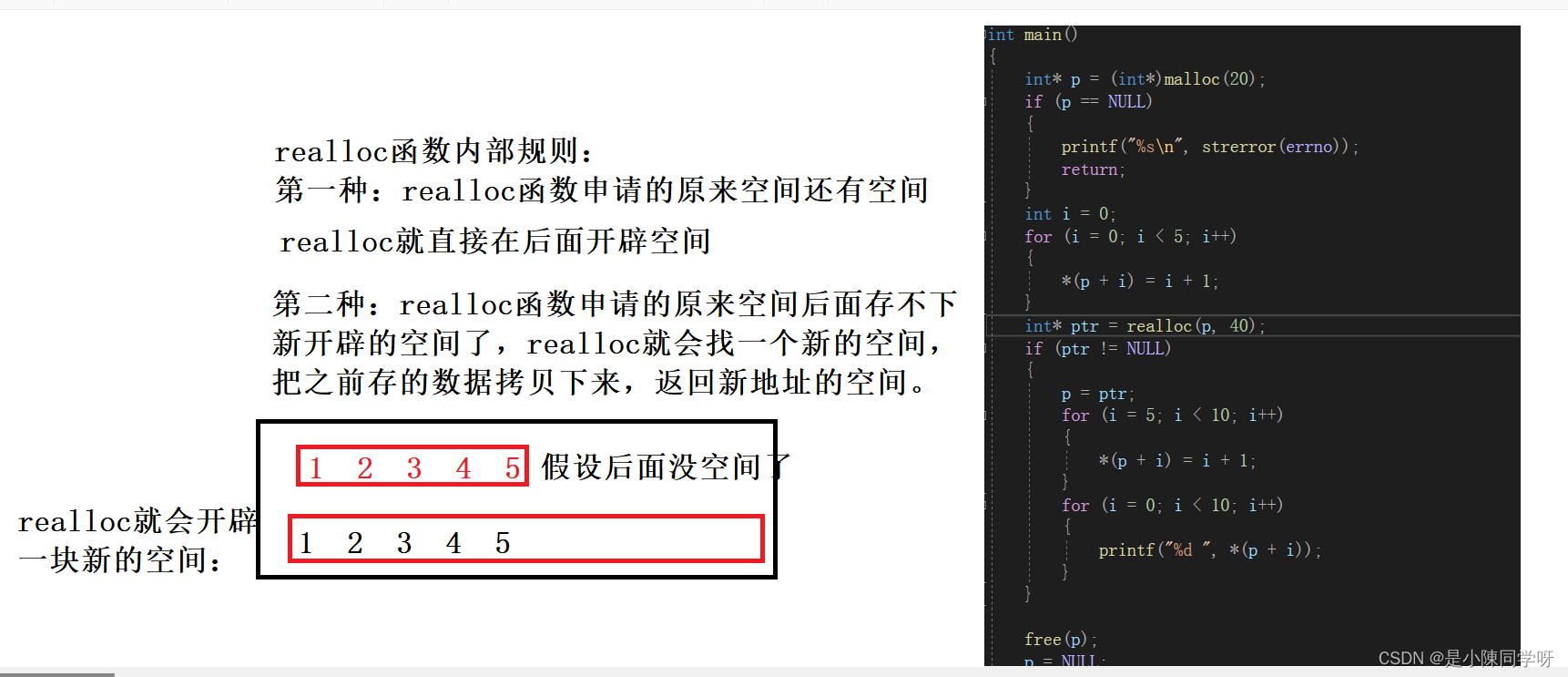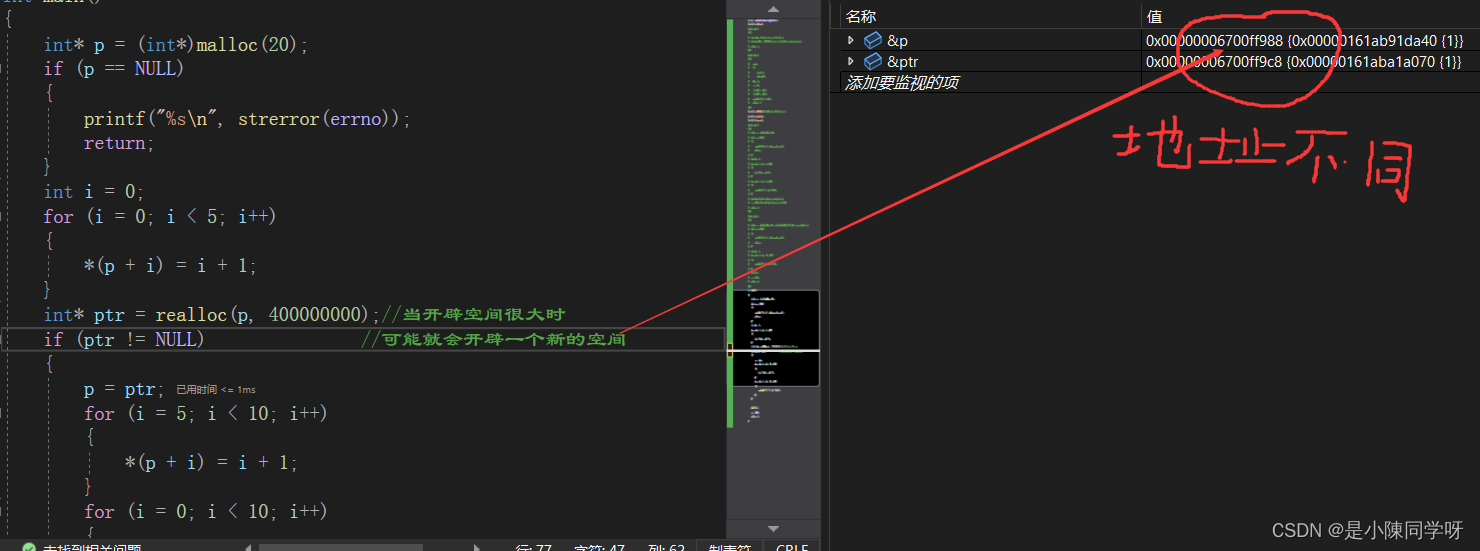1.realloc会找到更大的空间。

2.将原来的数据拷贝到新的空间。

3.释放新的空间。

4.返回新空间的地址。

realloc的使用：

``````int main()
{
int* p = (int*)malloc(20);
if (p == NULL)
{
printf("%s\n", strerror(errno));
return;
}
int i = 0;
for (i = 0; i < 5; i++)
{
*(p + i) = i + 1;
}
int* ptr = realloc(p, 40);
if (ptr != NULL)
{
p = ptr;
for (i = 5; i < 10; i++)
{
*(p + i) = i + 1;
}
for (i = 0; i < 10; i++)
{
printf("%d ", *(p + i));
}
}

free(p);
p = NULL;
return 0;
}``````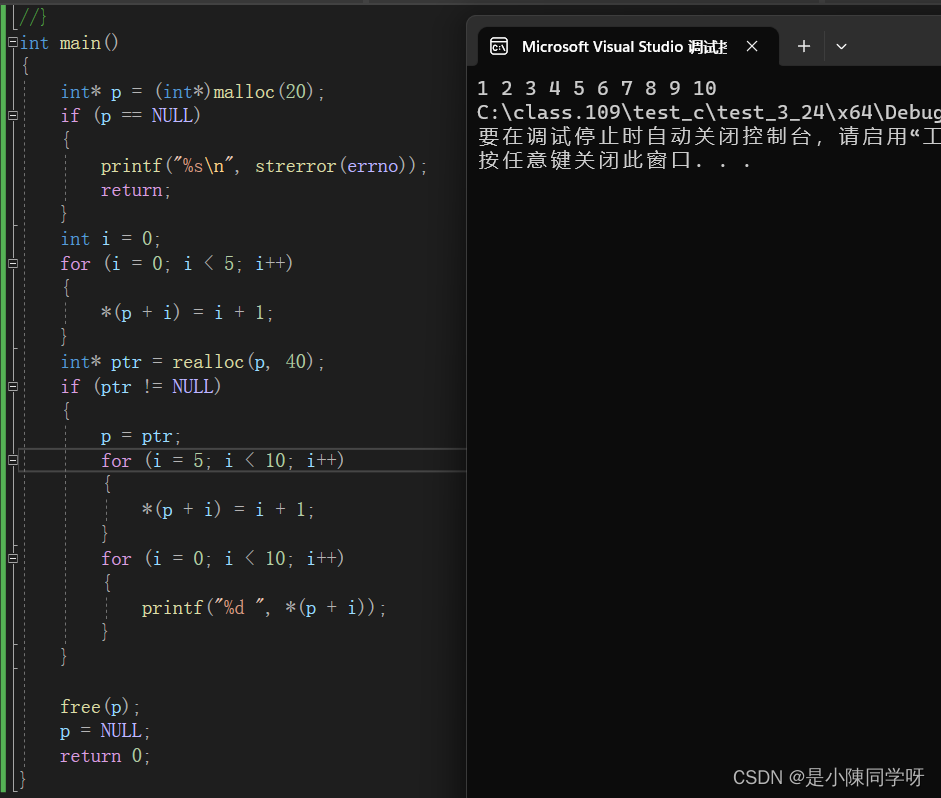# 三.常见的动态内存错误

### 1.对NULL指针的解引用

``````int main()
{
int* p = (int*)malloc(20);
int i = 0;
for (i = 0; i < 5; i++)
{
*(p + i) = i;
}
free(p);
p = NULL;
return 0;
}``````### 2.对动态开辟空间的越界访问

``````int main()
{
int* p = (int*)malloc(20);
if (p == NULL)
{
printf("%s\n", strerror(errno));
return;
}
int i = 0;
for (i = 0; i < 10; i++)
{
//上面只开辟了20个字节，但是这里要访问40个字节，就会越界访问
*(p + i) = i;
}
free(p);
p = NULL;

return 0;
}``````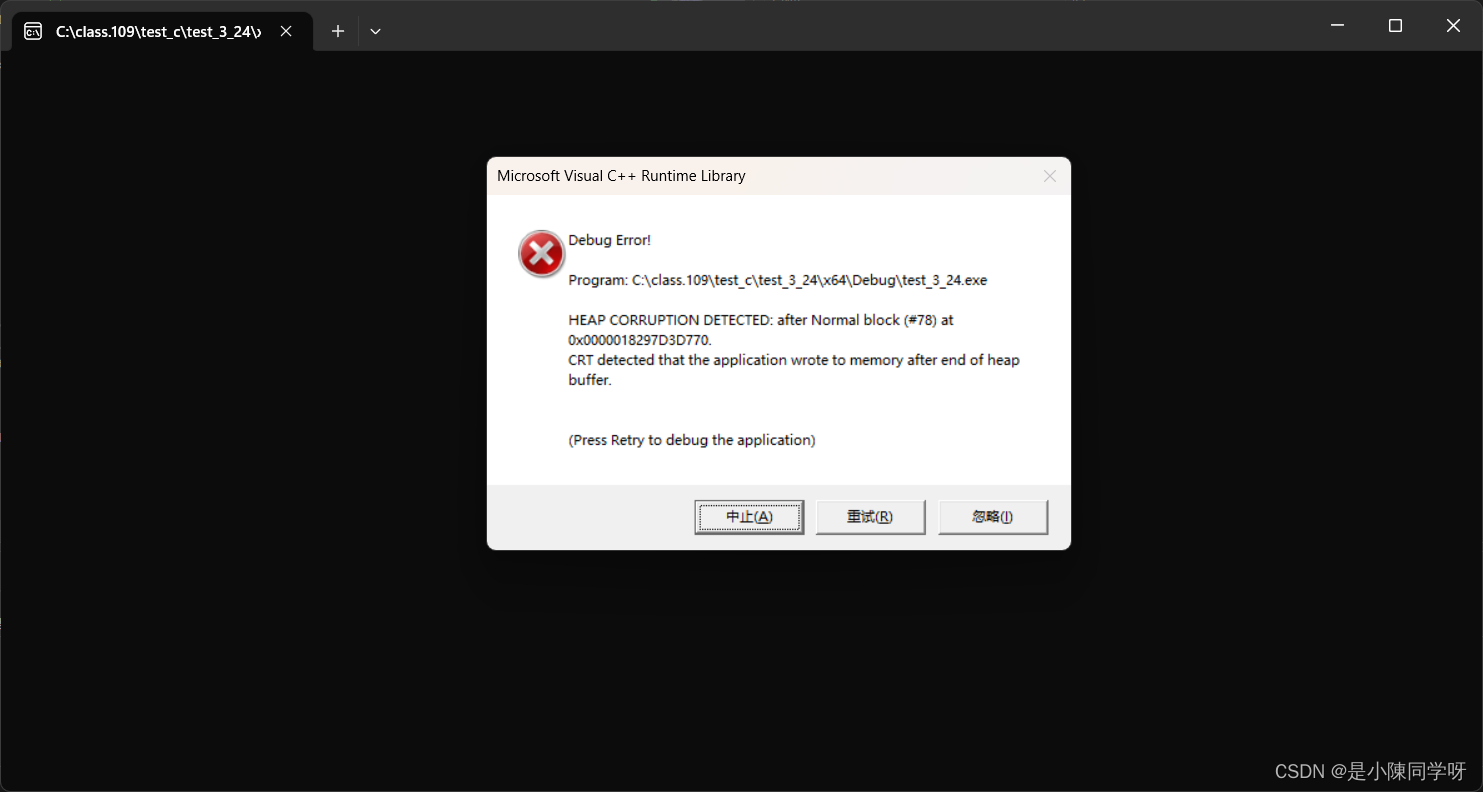### 3.对非动态开辟内存使用free释放

``````int main()
{
int arr = { 0 };
int* p = arr;
free(p);//这里的p是静态的，不能进行free
p = NULL;
return 0;
}``````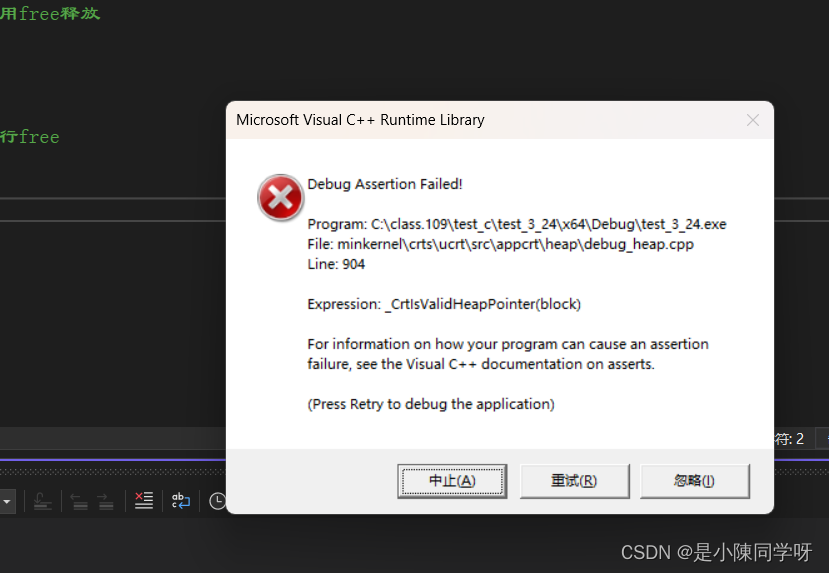### 5.对同一块动态内存多次释放

``````int main()
{
int* p = (int*)malloc(20);
if (p == NULL)
{
printf("%s\n", strerror(errno));
return;
}
free(p);//这里连续free了两次，会出错
free(p);
p = NULL;
return 0;
}``````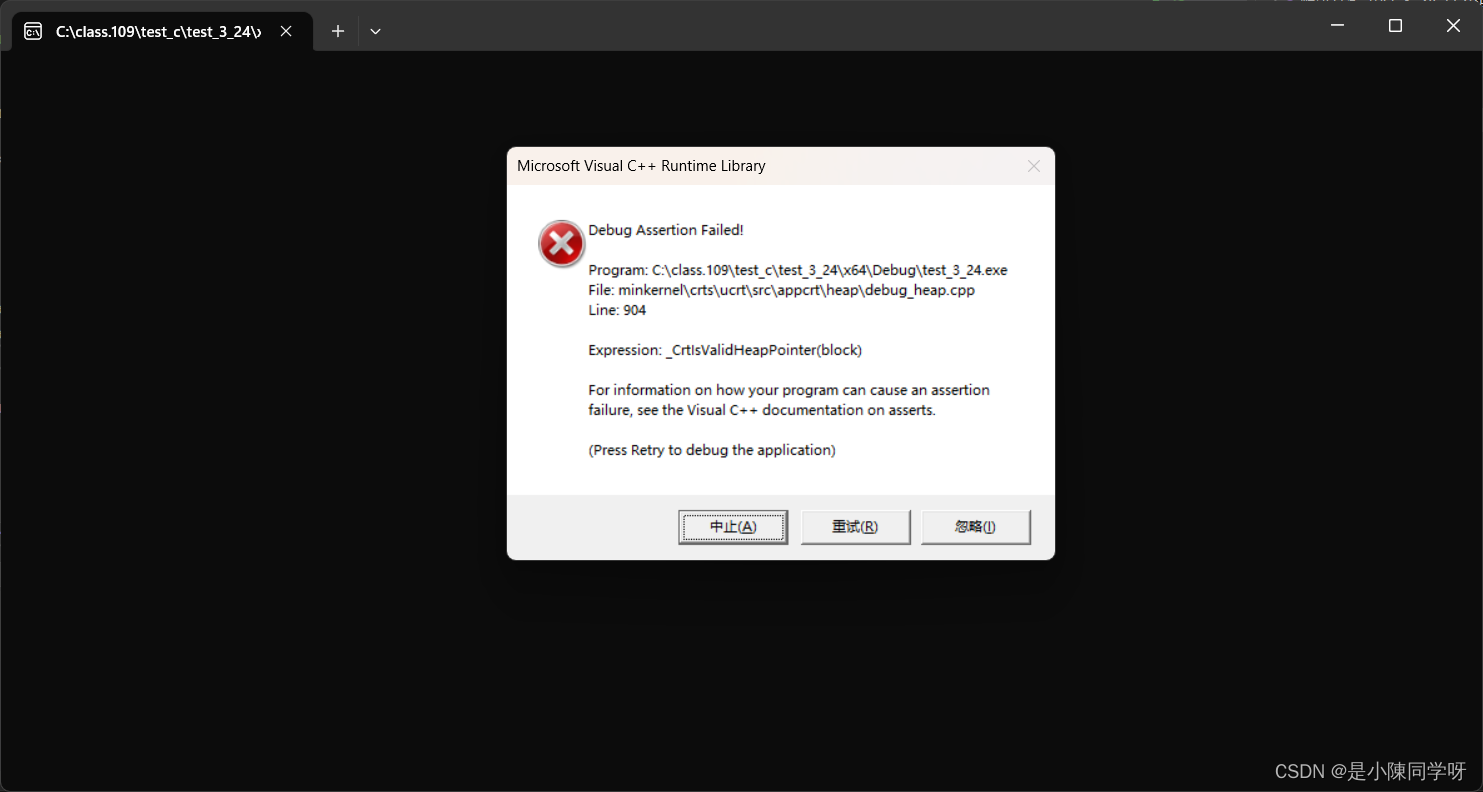如果是free了一次，把空间置为NULL之后，再次free，这是没有问题的。我们应当避免连续两次free同一块空间。

``````int main()
{
int* p = (int*)malloc(20);
if (p == NULL)
{
printf("%s\n", strerror(errno));
return;
}
free(p);//这样就是可行的
p = NULL;
free(p);
p = NULL;
return 0;
}``````

### 6.动态开辟内存忘记释放(内存泄漏)

``````int main()
{
int* p = (int*)malloc(20);
if (p == NULL)
{
printf("%s\n", strerror(errno));
return;
}
int i = 0;
for (i = 0; i < 5; i++)
{
*(p + i) = i;
}
//这里没有对内存进行free，就会造成内存泄漏
return 0;
}
``````

# 四.经典的几个面试题

``````void GetMemory(char* p)
{
p = (char*)malloc(100);
}

void Test(void)
{
char* str = NULL;
GetMemory(str);
strcpy(str, "hello world");
printf(str);
}
int main()
{
Test();
return 0;
}``````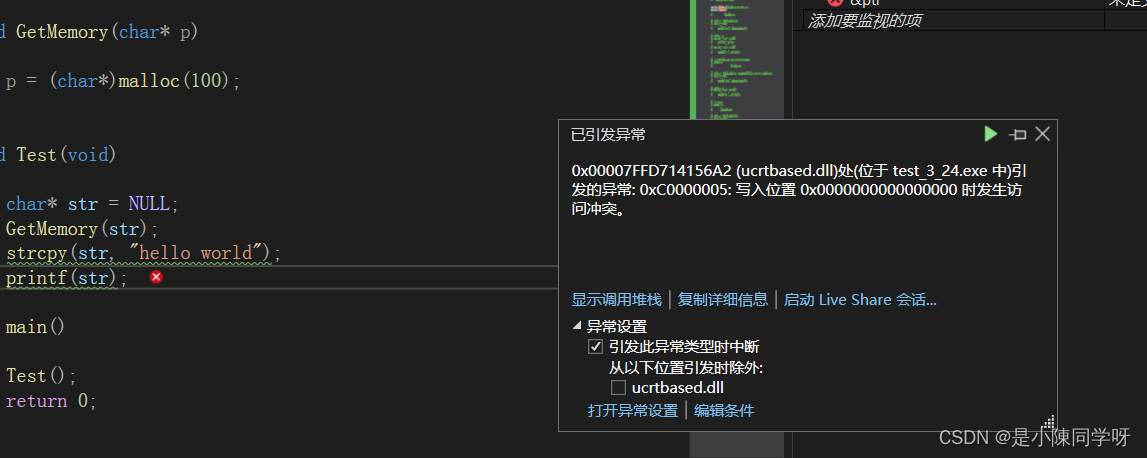1. 调用GetMemory函数的时候，str的传参为值传递，p是str的临时拷贝，所以在GetMemory函数内部讲动态开辟空间的地址存放在p中的时候，不会影响str。所以GetMemory函数返回之后，str中依然是NULL指针。strcpy函数就会调用失败，原因是对NULL的解引用操作,程序会崩溃。

2. GetMemory函数内容malloc申请的空间没有机会释放,造成了内存泄漏

``````void GetMemory(char** p)
{
*p = (char*)malloc(100);
}
void Test(void)
{
char* str = NULL;
GetMemory(&str);//使用传址调用
strcpy(str, "hello world");
printf(str);
free(str);//释放掉空间
str = NULL;
}
int main()
{
Test();
return 0;
}
``````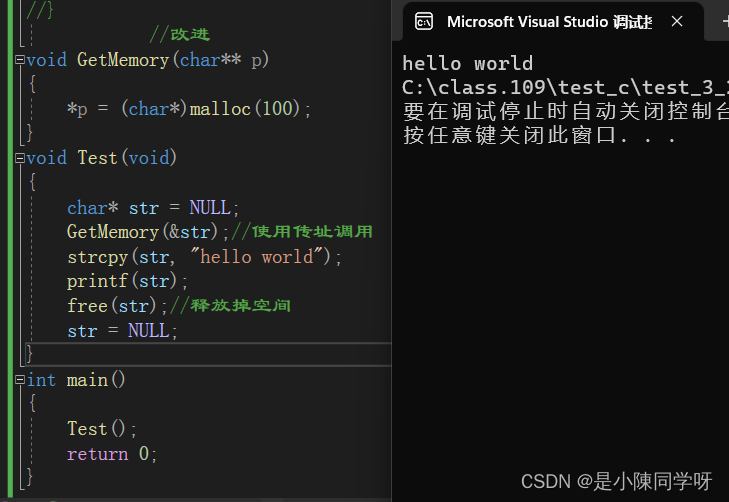``````char* GetMemory()
{
char p[] = "hello world";
return p;
}
void Test()
{
char* str = NULL;
str = GetMemory();
printf(str);
}
int main()
{
Test();
return 0;
}``````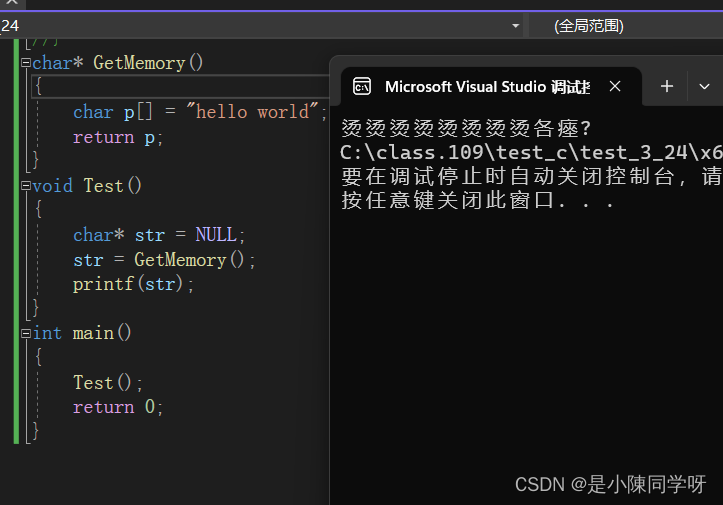GetMemory函数内部创建的数组是临时的，虽然返回了数组的起始地址给了str,但是数组的内存出了。GetMemory函数就被回收了，而str依然保存了数组的起始地址，这时如果使用str, str就是野指针。

``````char* GetMemory()
{
char *p = "hello world";//p是放在静态区的，只有整个程序退出时，才会销毁
return p;
}
void Test()
{
char* str = NULL;
str = GetMemory();
printf(str);
}
int main()
{
Test();
return 0;
}``````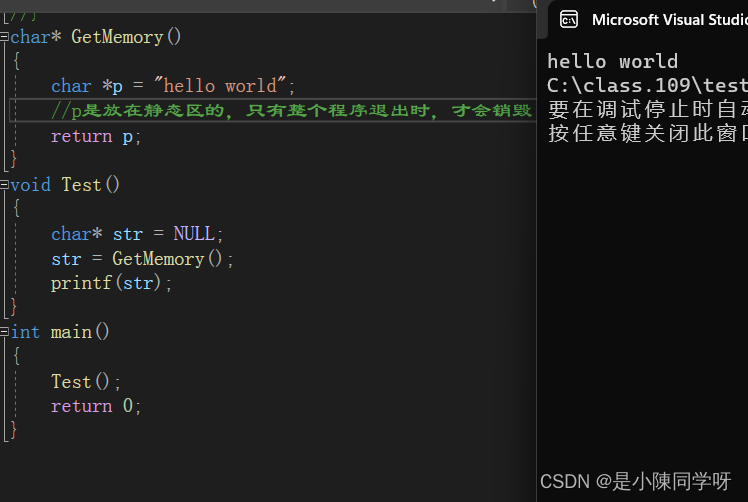### python编程：使用pyecharts绘制拟合曲线图

pyecharts库是python下实现的echarts图表绘制库&#xff0c;接下来&#xff0c;我们使用pyecharts来绘制一条曲线&#xff0c;来体验一下pyecharts的基本使用效果。 1、首先&#xff0c;我们要安装下pyecharts库&#xff0c;在pycharm终端输入安装命令&#xff1a; pip install…

### 乐观锁和悲观锁 面试题

Mysql的乐观锁和悲观锁 实现方式加锁时机常见的调用方式优势不足适用场景乐观锁开发自定义更新数据的时候sql语句中进行version的判断高并发容易出现不一致的问题高并发读&#xff0c;少写悲观锁Mysql内置查询数据的开始select * for update保证一致性低并发互联网高并发场景极…

### 有手就行 -- 搭建图床（PicGo+腾讯云）

&#x1f373;作者&#xff1a;贤蛋大眼萌&#xff0c;一名很普通但不想普通的程序媛\color{#FF0000}{贤蛋 大眼萌 &#xff0c;一名很普通但不想普通的程序媛}贤蛋大眼萌&#xff0c;一名很普通但不想普通的程序媛&#x1f933; &#x1f64a;语录&#xff1a;多一些不为什么的…

### async与await异步编程

ECMA2017中新加入了两个关键字async与await 简单来说它们是基于promise之上的的语法糖&#xff0c;可以让异步操作更加地简单明了 首先我们需要用async关键字&#xff0c;将函数标记为异步函数 async function f() {} f()异步函数就是指&#xff1a;返回值为promise对象的函…

### 银河麒麟v10sp2安装nginx

nginx官网下载&#xff1a;http://nginx.org/download/ 银河麒麟系统请先检查yum源是否配置&#xff0c;若没有配置请参考&#xff1a;https://qdhhkj.blog.csdn.net/article/details/129680789 一、安装 1、yum安装依赖 yum install gcc gcc-c make unzip pcre pcre-devel …

### 从 X 入门Pytorch——BN、LN、IN、GN 四种归一化层的代码使用和原理

Pytorch中四种归一化层的原理和代码使用前言1 Batch Normalization&#xff08;2015年提出&#xff09;Pytorch官网解释原理Pytorch代码示例2 Layer Normalization&#xff08;2016年提出&#xff09;Pytorch官网解释原理Pytorch代码示例3 Instance Normalization&#xff08;2…